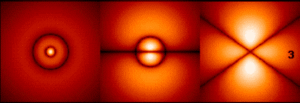Home » orbital angular momentum

# orbital angular momentum

« Back to Glossary Index

(Symbol: ɭ or L). Electrons have two types of rotational motion: orbital angular momentum and spin. Orbital angular momentum is a property of the electron’s rotational motion that is related to the shape of its orbital. The orbital is the region around the nucleus where the electron will be found if detection is undertaken. You may have seen the orbital described as the “electron cloud” around the nucleus.Electron orbitals in a hydrogen atom, diagrammed. All three images show the orbital of an electron at the same energy level but with differently shaped orbitals. The different shapes result in orbital angular momenta of different values.Source: https://commons.wikimedia.org/wiki/File:HAtomOrbitals.png

Orbital angular momentum is thought of as analogous to angular momentum in classical physics. The same symbol is used for both: ɭ or L. (There is no consensus as to the reason that L was chosen to symbolize angular momentum). While orbital angular momentum is thought of as paralleling angular momentum in classical physics, the meaning of the term in quantum physics cannot be easily explained in words. It can best be expressed mathematically. Short of the math, perhaps the best that can be done is to show a diagram and hope that something about it is communicated.

Other names. Orbital angular momentum is also called “extrinsic angular momentum” and the “Azimuthal quantum number.” Sometimes, people use the terms “spin” and “orbital angular momentum” interchangeably. That can be confusing because “spin” also names a completely different type of rotation of subatomic particles.

Conservation of angular momentum in quantum physics. Like angular momentum in classical physics, angular momentum in quantum physics is a conserved quantity. In quantum physics, the conserved quantity, however, is the total of orbital angular momentum plus spin. In other words, during interactions of particles, the sum of the orbital angular momentum plus spin starts at one number and must finish with that number.

Quantization of orbital angular momentum. In quantum physics, orbital angular momentum is quantized. It can take on only certain whole number values and cannot take on values in between these. It is not a continuous property but is treated as having discrete values.

Quantum numbers. Orbital angular momentum is one of the electron’s “Quantum Numbers,” or key properties. When orbital angular momentum is thought of as being a Quantum Number, it is called the “Azimuthal quantum number.” Often, physicists list four key quantized properties of the electron as its “Quantum Numbers”:

1. Principal quantum number (energy level of the electron)
2. Azimuthal quantum number (orbital angular momentum)
3. Magnetic quantum number
4. Spin quantum number

Each of these numbers is quantized; that is, their values can be only whole numbers. Mass doesn’t appear as a Quantum Number because it is not quantized.

This site uses Akismet to reduce spam. Learn how your comment data is processed.